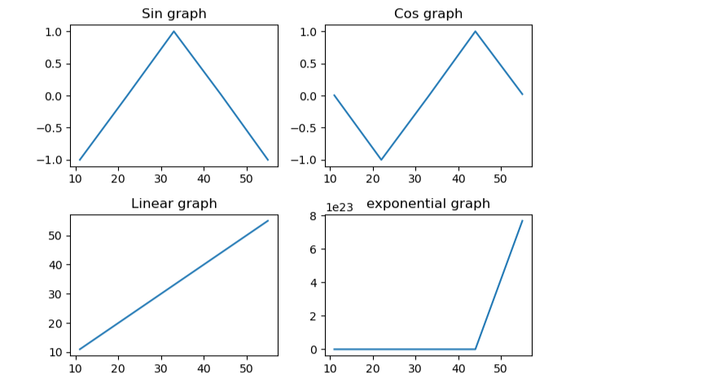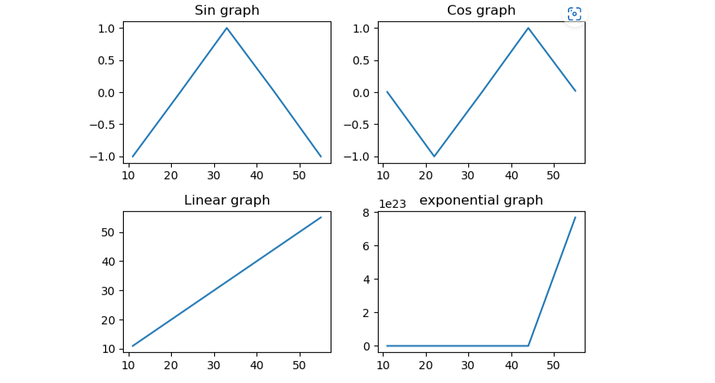# How to Add Title to Subplots in Matplotlib?

Matplotlib is a Python package that is widely used for the purpose of creating plots, subplots, and visualizations with titles and descriptions. When making more than one subplot, it can be helpful to give each one a title to give the user and the viewer more context and clarity. This article will show how to give a subplot a title in Matplotlib.

## Matplotlib

Matplotlib is a plotting library for the Python programming language and NumPy, its extension for numerical mathematics. Tkinter, wxPython, Qt, and GTK GUI toolkits may include diagrams utilizing its object-oriented API.

The matplotlib.pyplot is a collection of command-style methods that allow matplotlib to function similarly to MATLAB. Each pyplot function alters a figure in some manner, whether it is by adding a plotting area, plotting lines, adding labels, etc. The current figure and plotting area are saved between function calls in matplotlib.pyplot, and the plotting functions are always applied to the active set of axes (please note that the term "axes" here and throughout the documentation refers to the axes component of a figure and not the mathematical term for more than one axis).

## Subplots

Subplots in Matplotlib allow multiple plots or charts to be displayed within a single figure. We can compare and analyze multiple sets of data at the same time with the help of subplots. This makes spotting or identifying trends, patterns, and relationships easier.

A subplot is a grid of smaller plots that are part of a larger plot. Each subplot has its own place in the grid, which is based on the number of rows and columns in the grid and where the subplot is in that grid.

Matplotlib’s “subplots” method lets us create subplots. This function returns a figure object and an array of subplot objects. We can plot our data in each subplot by using these subplot objects.

### Syntax

fig,ax=plt.subplots(nrows,ncolumns,index)


### Explanation

• nrows − This parameter specifies the number of rows of subplots in the grid.

• ncolumns − This parameter specifies the number of columns of subplots in the grid.

• index − This parameter specifies the index of the current subplot. The index starts at 1 and increases row-wise.

There are mainly two ways using which we can add titles to subplots, they are −

• set_title() function

• title.set_text() function

## set_title() Function

The set_title() function is present in the matplotlib library of Python, used to set titles for the plots and subplots.

### Syntax

axes.set_title(label,font_dict=None,Loc=None,pad=None,*,y=None)


### Explanation

• Axes − It is the axes of the object.

• label (values: str) − It defines the text that we want to use as a title for the subplot.

• font_dict − font_dict is a dictionary of font properties to apply to the title text.

• loc − It is a string or integer that specifies the location of the title. Common arguments that we can pass our ‘center’,’ left’,’ right’, etc.

• pad − It is the padding between the title and the top of the plot.

### Example 1

# importing all the important packages
import numpy as n
import matplotlib.pyplot as p

# create array data using whuich we will plot the graph
x=n.array([11, 22,33, 44, 55])

# making subplots
f, a = p.subplots(2, 2)

# set data with subplots and plot
a[0, 0].plot(x, n.sin(x))
a[0, 1].plot(x, n.cos(x))
a[1, 0].plot(x, x)
a[1, 1].plot(x, n.exp(x))

# set the title to all subplots
a[0, 0].set_title("Sin graph")
a[0, 1].set_title("Cos graph")
a[1, 0].set_title("Linear graph")
a[1, 1].set_title("exponential graph")

# set spacing
f.tight_layout()
p.show()


### Output## title.set_text() function

The Matplotlib set_text() function is a text class function used to set the text of a title or other text object in a subplot or plot. The set_text() function can be used to dynamically alter the text of a title or other text object in response to data or user input changes.

When adding a title to a plot or a subplot, the set_text() function is usually used with the get_title() function, which is a method of the Axes class and is used to get the title object for the current subplot.

t_obj.set_text(Text_s)

• t_obj: is a text object such as a label or title.

• Text_s: is the text to set.

### Example 2

# importing all the important packages
import numpy as n
import matplotlib.pyplot as p

# create array data using whuich we will plot the graph
x=n.array([11, 22,33, 44, 55])

# making subplots
f, a = p.subplots(2, 2)

# set data with subplots and plot
a[0, 0].plot(x, n.sin(x))
a[0, 1].plot(x, n.cos(x))
a[1, 0].plot(x, x)
a[1, 1].plot(x, n.exp(x))

# set the title to all subplots
a[0, 0].title.set_text("Sin graph")
a[0, 1].title.set_text("Cos graph")
a[1, 0].title.set_text("Linear graph")
a[1, 1].title.set_text("exponential graph")

# set spacing
f.tight_layout()
p.show()


### Output## Conclusion

In conclusion, adding titles to the subplots in Matplotlib can be useful for providing more clarity and context to the viewer, to add a title to a subplot we have used two methods or functions that are available in the matplotlib library. By following the steps outlined in this article, you can easily add titles to subplots in Matplotlib using Python.

Updated on: 31-May-2023

2K+ Views